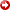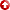[First Hit]Sergio Gamaliel Perez1

(1) P.D.V.S.A, Maracaibo, Venezuela

ABSTRACT: Computer simulation of hanging wall deformation

A computer simulation based upon a finite difference model of hanging wall deformation is elaborated via Mathematica programming.

Vertical simple shear is usually adapted to set up the boundary conditions because it facilitates the calculations. Nevertheless, in the program is used a Mathematica(r)-built displacement direction function consistent with inclined simple shear conditions.

To find the derivatives of the displacement direction function, an essential sub-routine in the simulation, two approaches are used. The first involves a numerical solution based upon the Crank-Nicholson method. The second requires thecalculusof the derivatives with the support of Mathematica(r) built-in functions.

A brief analysis is carried out on the algorithms devised for these sub-routines and on its graphical display.

An assesment on the efficiency of Mathematica(r) for linear finite-element modelling is given based upon the availability and efficiency of resources found in the system during the programming of the algorithms.

The graphical and analytical capabilities of Mathematica(r) are used to generate the trajectories and velocity fields of the hanging wall particles with respect to an Eulerian system of reference.

The final outcome of the simulation is presented as an animated sequence. It comprises the visual display of syntectonic sedimentation during listric faulting,and later on, partial inversion with thrusting.